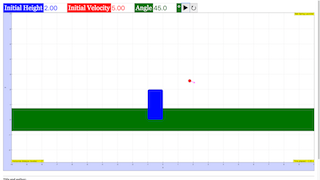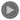Intro Page

Projectile Motion: Experiment and Computational Model

Developed by Todd Zimmerman

Connecting computation to experiment is at the heart of physics. This set of exercises requires students to create a computational model for a ball launched into the air to determine the distance traveled and total time in the air. The students then must perform the experiment by launching the ball with a spring launcher. Data from the experiment must be entered into the computational model and the experimental results are compared to the computer model.

 Subject Areas Mechanics and Experimental Labs First Year IPython/Jupyter Notebook and Sage Worksheet Explain that the time an object is in the air depends only on motion in the y-direction (Exercise 4) Relate the initial velocity of a launched ball to the horizontal velocity when the ball is launched horizontally (Exercise 2) Make use of the fact that horizontal and vertical motion are independent to solve a 2D motion problem by breaking problem up into two 1D problems (Exercise 1) Convert equations of motion into a computational model (program) with discrete time-steps (Exercise 1) Use experimental data as an input into a computational model (Exercise 6) Explain the limitations of computational models in predicting experimental results (Exercise 6) 180 min
ball_launch_lab_activity(Exercise 1).ipynb

#Exercise 1

from vpython import *

from math import *

import matplotlib.pyplot as plt

%matplotlib inline

scene=canvas(title='Ball Spring Launcher') #Sets title for graphics window

scene.range=5 #Determines how wide graphics window is (sorta)

scene.center=vector(0,0,0)

dt = 0.01 #dt is the time step 'Delta t'

t=0 #Set initial time to zero

g = vector(0,-9.8,0)

h = 2 #initial height of ball

v_init=10

ball = sphere(pos=vector(0,h,0), radius=.2, color=color.red,make_trail=True) #Create sphere that will appear on screen

ground = box(pos=vector(0,0,0),color=color.green,size=vector(10,.1,5)) #Create green "grass" to give a reference frame

table = box(pos=vector(-0.5,h/2,0), color=color.blue, size=vector(1,h,1)) #Create a "table" for reference

ball.m = 1 #Mass of ball

ball.v = vector(v_init,0,0) #Initial velocity vector of ball

scene.waitfor("click") #Don't go onto the next step until you have clicked on the screen

while ball.pos.y>0: #Stop when ball hits floor

rate(40) #Determines how quickly program runs (roughly 30 frames per second)

ball.pos = ball.pos + ball.v*dt #Update position of ball

ball.v = ball.v + g*dt #Update velocity of ball

t+=dt #Calculate total time elapsed

print("Time elapsed = ", t)

print("Horizontal distance traveled = ", ball.pos.x)

Exercise 1

### EXERCISE 1

Create a computational model of ball launched with some velocity in the horizontal direction. Create a visual representation of the motion (either 2D animation or a graph of the 2D trajectory.

Your model should include the mass and size of the ball, the initial horizontal speed, and the initial height of the ball above the floor. The model should stop when the ball hits the floor. The model should return the horizontal distance the ball travels before hitting the floor as well as the total time it is in the air.

The equations that model the motion of the ball are

$\begin{array}{}\text{(3)}& \frac{d\stackrel{\to }{r}}{dt}=\stackrel{\to }{v}\end{array}$

and

$\begin{array}{}\text{(4)}& \frac{d\stackrel{\to }{v}}{dt}=\frac{\stackrel{\to }{F}}{m}.\end{array}$

These equations involve derivatives but computer models must use time steps that have a finite size (called discrete time steps). In terms of finite differences these two equations can be written as

$\begin{array}{}\text{(5)}& \frac{\mathrm{\Delta }\stackrel{\to }{r}}{\mathrm{\Delta }t}=\stackrel{\to }{v}\end{array}$

and

$\begin{array}{}\text{(6)}& \frac{\mathrm{\Delta }\stackrel{\to }{v}}{\mathrm{\Delta }t}=\frac{\stackrel{\to }{F}}{m}.\end{array}$

Remember that the $\mathrm{\Delta }$ symbol means “change in …” so $\mathrm{\Delta }t$ is the “change in time”. Use these equations to find two equations that allow you to update the position and velocity of the particle at each time step.

Hint: Remember that you have motion along the x-axis and motion along the y-axis.

Exercise 3

### EXERCISE 3

Modify your code so you can vary the angle at which the ball is launched in addition to the launch speed.

ball_launch_lab_activity(Exercise 3).ipynb

#Exercise 3

from vpython import *

from math import *

scene=canvas(title='Ball Spring Launcher') #Sets title for graphics window

scene.range=5 #Determines how wide graphics window is (sorta)

scene.center=vector(0,0,0)

dt = 0.01 #dt is the time step 'Delta t'

t=0 #Set initial time to zero

g = vector(0,-9.8,0)

h = 2 #initial height of ball

theta=pi/4

v_init=5

v=vector(v_init*cos(theta),v_init*sin(theta),0)

ball = sphere(pos=vector(0,h,0), radius=.2, color=color.red, make_trail=True) #Create sphere that will appear on screen

ground = box(pos=vector(0,0,0),color=color.green,size=vector(10,.1,5)) #Create green "grass" to give a reference frame

table = box(pos=vector(-0.5,h/2,0), color=color.blue, size=vector(1,h,1)) #Create a "table" for reference

ball.m = 1 #Mass of ball

ball.v = v #Initial momentum vector of ball

scene.waitfor("click") #You must click on the image before it will start moving

while ball.pos.y>0+ball.radius: #Stop when ball hits floor

rate(40) #Determines how quickly program runs (roughly 30 frames per second)

ball.pos = ball.pos + ball.v*dt #Update position of ball

ball.v = ball.v + g*dt #Update momentum of ball

t+=dt #Calculate total time elapsed

print("Time elapsed = ", t)

print("Horizontal distance traveled = ", ball.pos.x)

### Translations

Code Language Translator Run### Software Requirements

SoftwareRequirements

 Android iOS Windows MacOS with best with Chrome Chrome Chrome Chrome support full-screen? Yes. Chrome/Opera No. Firefox/ Samsung Internet Not yet Yes Yes cannot work on some mobile browser that don't understand JavaScript such as..... cannot work on Internet Explorer 9 and below

### CreditsFremont Teng; Loo Kang Wee; based on codes by Todd Zimmerman

### Other resources

1. http://www.compadre.org/Physlets/mechanics/illustration3_4.cfm Illustration 3.4: Projectile Motion by W. Christian and M. Belloni
2. http://physics.weber.edu/amiri/director-dcrversion/newversion/airresi/AirResi_1.0.html Trajectory of a ball with air resistance by Farhang Amiri
3. http://www.walter-fendt.de/html5/phen/projectile_en.htm HTML5 version of Projectile Motion by Walter Fendt
4. http://www.compadre.org/OSP/items/detail.cfm?ID=7299&S=7 Ejs Intro 2DMotionLab Model by  Anne Cox, Wolfgang Christian, and Mario Belloni
5. http://www.phy.ntnu.edu.tw/ntnujava/index.php?topic=623.0 Projectile motion with equations by Fu-Kwun Hwang
6. http://www.phy.ntnu.edu.tw/ntnujava/index.php?topic=1832.0 Airdrag by Fu-Kwun Hwang and ahmedelshfie
8. http://ophysics.com/k8.html by This email address is being protected from spambots. You need JavaScript enabled to view it.
9. http://ophysics.com/k9.html by This email address is being protected from spambots. You need JavaScript enabled to view it.

# Testimonials (0)

There are no testimonials available for viewing. Login to deploy the article and be the first to submit your review!